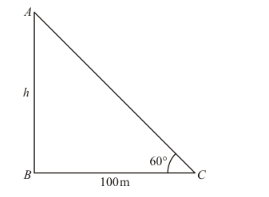# If the angle of elevation of a tower from a distance of 100 metres from its foot is 60°

Question:

If the angle of elevation of a tower from a distance of 100 metres from its foot is 60°, then the height of the tower is

(a) $100 \sqrt{3} \mathrm{~m}$

(b) $\frac{100}{\sqrt{3}} m$

(c) $50 \sqrt{3}$

(d) $\frac{200}{\sqrt{3}} m$

Solution:

Letbe the height of tower ismetersGiven that: angle of elevation isfrom tower of foot and distancemeters.

Here, we have to find the height of tower.

So we use trigonometric ratios.

In a triangle,

$\Rightarrow \tan C=\frac{A B}{B C}$

$\Rightarrow \tan 60^{\circ}=\frac{A B}{B C}$

$\Rightarrow \sqrt{3}=\frac{h}{100}$

$\Rightarrow h=100 \sqrt{3}$

Hence correct option is a.